# How to subtract fractions with different denominators

Want to find out how to subtract fractions with different denominators? We've provided a step-by-step guide of how to subtract fractions with different denominators to teach you everything you need to know.## Step 1: Find the least common denominator

Find the least common denominator (LCD) of the fractions. The LCD is the denominators’ least common multiple.

## Step 2: Find the equivalent fraction

Find the equivalent fraction of each fraction so that they all have the LCD as the denominator.

## Step 3: Subtract the new numerators

Subtract the new numerators and write the LCD as the denominator.

## Step 4: Simplify if necessary

Reduce the difference to its lowest terms.

## Examples of how to subtract fractions with different denominators

Q1) Find the difference of the following sets of fractions: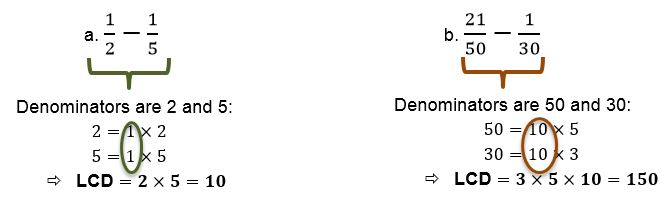Express the fractions with their equivalents so they share common denominators using the LCD.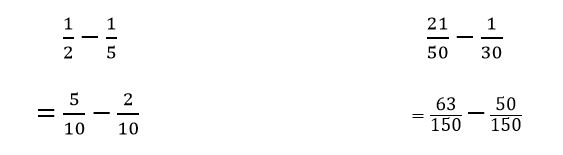Subtract the numerators and write down the LCD as the denominator.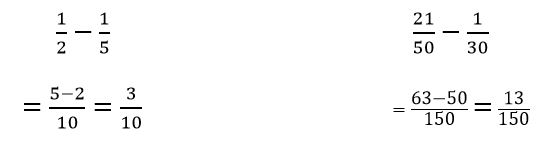Check if the difference is already reduced to its lowest terms.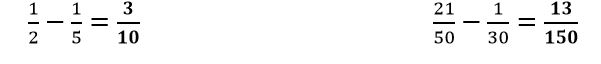Q2) Jonathan needs to cover 3/4 mile as part of his daily run. If he has already covered 5/12 miles, how far must he need to cover to meet his required distance?

We want to find the difference of 3/4 and 5/12 to determine the remaining distance.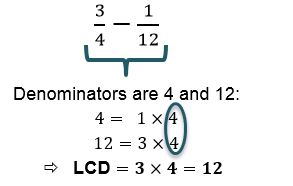Express the fractions so they have 12 as their denominator.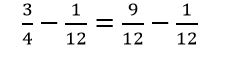Subtract the numerators to find the difference.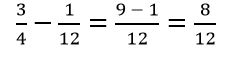Simplify to find the final answer.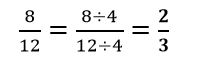This means that Jonathan needs to cover 2/3 mile more to meet his practice requirement.

#### Join today

The fastest way to practice

Unlock our complete testing platform and improve faster that ever.